Discrete Random Variables

# Hypergeometric Distribution

There are five characteristics of a hypergeometric experiment.

1. You take samples from two groups.
2. You are concerned with a group of interest, called the first group.
3. You sample without replacement from the combined groups. For example, you want to choose a softball team from a combined group of 11 men and 13 women. The team consists of ten players.
4. Each pick is not independent, since sampling is without replacement. In the softball example, the probability of picking a woman first is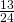. The probability of picking a man second is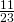if a woman was picked first. It is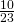if a man was picked first. The probability of the second pick depends on what happened in the first pick.
5. You are not dealing with Bernoulli Trials.

The outcomes of a hypergeometric experiment fit a hypergeometric probability distribution. The random variable X = the number of items from the group of interest.

A candy dish contains 100 jelly beans and 80 gumdrops. Fifty candies are picked at random. What is the probability that 35 of the 50 are gumdrops? The two groups are jelly beans and gumdrops. Since the probability question asks for the probability of picking gumdrops, the group of interest (first group) is gumdrops. The size of the group of interest (first group) is 80. The size of the second group is 100. The size of the sample is 50 (jelly beans or gumdrops). Let X = the number of gumdrops in the sample of 50. X takes on the values x = 0, 1, 2, …, 50. What is the probability statement written mathematically?

P(x = 35)

Try It

A bag contains letter tiles. Forty-four of the tiles are vowels, and 56 are consonants. Seven tiles are picked at random. You want to know the probability that four of the seven tiles are vowels. What is the group of interest, the size of the group of interest, and the size of the sample?

Suppose a shipment of 100 DVD players is known to have ten defective players. An inspector randomly chooses 12 for inspection. He is interested in determining the probability that, among the 12 players, at most two are defective. The two groups are the 90 non-defective DVD players and the 10 defective DVD players. The group of interest (first group) is the defective group because the probability question asks for the probability of at most two defective DVD players. The size of the sample is 12 DVD players. (They may be non-defective or defective.) Let X = the number of defective DVD players in the sample of 12. X takes on the values 0, 1, 2, …, 10. X may not take on the values 11 or 12. The sample size is 12, but there are only 10 defective DVD players. Write the probability statement mathematically.

P(x ≤ 2)

Try It

A gross of eggs contains 144 eggs. A particular gross is known to have 12 cracked eggs. An inspector randomly chooses 15 for inspection. She wants to know the probability that, among the 15, at most three are cracked. What is X, and what values does it take on?

You are president of an on-campus special events organization. You need a committee of seven students to plan a special birthday party for the president of the college. Your organization consists of 18 women and 15 men. You are interested in the number of men on your committee. If the members of the committee are randomly selected, what is the probability that your committee has more than four men?

This is a hypergeometric problem because you are choosing your committee from two groups (men and women).

a. Are you choosing with or without replacement?

a. without

b. What is the group of interest?

b. the men

c. How many are in the group of interest?

c. 15 men

d. How many are in the other group?

d. 18 women

e. Let X = _________ on the committee. What values does X take on?

e. Let X = the number of men on the committee. x = 0, 1, 2, …, 7.

f. The probability question is P(_______).

f. P(x > 4)

Try It

A palette has 200 milk cartons. Of the 200 cartons, it is known that ten of them have leaked and cannot be sold. A stock clerk randomly chooses 18 for inspection. He wants to know the probability that among the 18, no more than two are leaking. Give five reasons why this is a hypergeometric problem.

### Notation for the Hypergeometric: H = Hypergeometric Probability Distribution Function

X ~ H(r, b, n)

Read this as “X is a random variable with a hypergeometric distribution.” The parameters are r, b, and n; r = the size of the group of interest (first group), b = the size of the second group, n = the size of the chosen sample.

A school site committee is to be chosen randomly from six men and five women. If the committee consists of four members chosen randomly, what is the probability that two of them are men? How many men do you expect to be on the committee?

Let X = the number of men on the committee of four. The men are the group of interest (first group).

X takes on the values 0, 1, 2, 3, 4, where r = 6, b = 5, and n = 4. X ~ H(6, 5, 4)

Find P(x = 2). P(x = 2) = 0.4545 (calculator or computer)

NOTE

Currently, the TI-83+ and TI-84 do not have hypergeometric probability functions. There are a number of computer packages, including Microsoft Excel, that do.

The probability that there are two men on the committee is about 0.45.

The graph of X ~ H(6, 5, 4) is: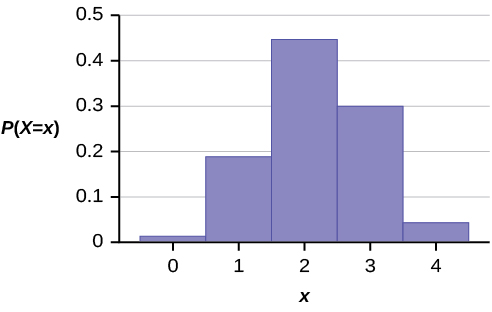The y-axis contains the probability of X, where X = the number of men on the committee.

You would expect m = 2.18 (about two) men on the committee.

The formula for the mean is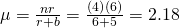Try It

An intramural basketball team is to be chosen randomly from 15 boys and 12 girls. The team has ten slots. You want to know the probability that eight of the players will be boys. What is the group of interest and the sample?

### Chapter Review

A hypergeometric experiment is a statistical experiment with the following properties:

1. You take samples from two groups.
2. You are concerned with a group of interest, called the first group.
3. You sample without replacement from the combined groups.
4. Each pick is not independent, since sampling is without replacement.
5. You are not dealing with Bernoulli Trials.

The outcomes of a hypergeometric experiment fit a hypergeometric probability distribution. The random variable X = the number of items from the group of interest. The distribution of X is denoted X ~ H(r, b, n), where r = the size of the group of interest (first group), b = the size of the second group, and n = the size of the chosen sample. It follows that
nr + b. The mean of X is μ =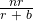and the standard deviation is σ =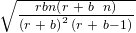.

### Formula Review

X ~ H(r, b, n) means that the discrete random variable X has a hypergeometric probability distribution with r = the size of the group of interest (first group), b = the size of the second group, and n = the size of the chosen sample.

X = the number of items from the group of interest that are in the chosen sample, and X may take on the values x = 0, 1, …, up to the size of the group of interest. (The minimum value for X may be larger than zero in some instances.)

nr + b

The mean of X is given by the formula μ =and the standard deviation is =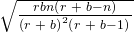.

Use the following information to answer the next five exercises: Suppose that a group of statistics students is divided into two groups: business majors and non-business majors. There are 16 business majors in the group and seven non-business majors in the group. A random sample of nine students is taken. We are interested in the number of business majors in the sample.

In words, define the random variable X.

X = the number of business majors in the sample.

X ~ _____(_____,_____)

<!– <solution id=”fs-idm46774464″> H(16, 7, 9) –>

What values does X take on?

2, 3, 4, 5, 6, 7, 8, 9

Find the standard deviation.

<!– <solution id=”fs-idm7562448″>

–>

On average (μ), how many would you expect to be business majors?

6.26

### HOMEWORK

A group of Martial Arts students is planning on participating in an upcoming demonstration. Six are students of Tae Kwon Do; seven are students of Shotokan Karate. Suppose that eight students are randomly picked to be in the first demonstration. We are interested in the number of Shotokan Karate students in that first demonstration.

1. In words, define the random variable X.
2. List the values that X may take on.
3. Give the distribution of X. X ~ _____(_____,_____)
4. How many Shotokan Karate students do we expect to be in that first demonstration?

<!– <solution id=”fs-idp3312224″> X = the number of Shotokan Karate students in the first demonstration 0, 1, 2, 3, 4, 5, 6, 7 X ~ H(7, 6, 8) 4.31 –>

In one of its Spring catalogs, L.L. Bean® advertised footwear on 29 of its 192 catalog pages. Suppose we randomly survey 20 pages. We are interested in the number of pages that advertise footwear. Each page may be picked at most once.

1. In words, define the random variable X.
2. List the values that X may take on.
3. Give the distribution of X. X ~ _____(_____,_____)
4. How many pages do you expect to advertise footwear on them?
5. Calculate the standard deviation.
1. X = the number of pages that advertise footwear
2. 0, 1, 2, 3, …, 20
3. X ~ H(29, 163, 20); r = 29, b = 163, n = 20
4. 3.03
5. 1.5197

Suppose that a technology task force is being formed to study technology awareness among instructors. Assume that ten people will be randomly chosen to be on the committee from a group of 28 volunteers, 20 who are technically proficient and eight who are not. We are interested in the number on the committee who are not technically proficient.

1. In words, define the random variable X.
2. List the values that X may take on.
3. Give the distribution of X. X ~ _____(_____,_____)
4. How many instructors do you expect on the committee who are not technically proficient?
5. Find the probability that at least five on the committee are not technically proficient.
6. Find the probability that at most three on the committee are not technically proficient.

<!– <solution id=”eip-idm90541104″> X = the number of people on the committee who are not technically proficient 0, 1, 2, 3, 4, 5, 6, 7, 8, 9, 10 X ~ B ( 10,  8 28 ) 2.8571 0.1269 0.6873 –>

Suppose that nine Massachusetts athletes are scheduled to appear at a charity benefit. The nine are randomly chosen from eight volunteers from the Boston Celtics and four volunteers from the New England Patriots. We are interested in the number of Patriots picked.

1. In words, define the random variable X.
2. List the values that X may take on.
3. Give the distribution of X. X ~ _____(_____,_____)
4. Are you choosing the nine athletes with or without replacement?
1. X = the number of Patriots picked
2. 0, 1, 2, 3, 4
3. X ~ H(4, 8, 9)
4. Without replacement

A bridge hand is defined as 13 cards selected at random and without replacement from a deck of 52 cards. In a standard deck of cards, there are 13 cards from each suit: hearts, spades, clubs, and diamonds. What is the probability of being dealt a hand that does not contain a heart?

1. What is the group of interest?
2. How many are in the group of interest?
3. How many are in the other group?
4. Let X = _________. What values does X take on?
5. The probability question is P(_______).
6. Find the probability in question.
7. Find the (i) mean and (ii) standard deviation of X.

<!– <solution id=”fs-idp25943152″> Cards that are not hearts 52 − 13 = 39 Other group = hearts; there are 13 hearts in a deck. X = the number of cards that are not hearts; x = 0, 1, 2, 3, … , 13 P(x = 13) ( 39 52 )( 38 51 )( 37 50 )( 36 49 )( 35 48 )( 34 47 )( 33 46 )( 32 45 )( 31 44 )( 30 43 )( 29 42 )( 28 41 )( 27 40 ) ≈ 0.0128 Mean = μ = nr r + b = (13)(39) 39 + 13 = 9.75 Standard Deviation = σ = rbn(r + b−n) (r + b) 2 (r + b−1) = 39(13)13(39 + 13–13) (39 + 13) 2 (39 + 13–1) ≈ 1.3653 Alternate Solution Set Up Cards that are hearts 13 Other group = non-hearts; b = 39 X = the number of cards that are hearts; x = 0, 1, 2, 3, … , 13 P(x = 0)

### Glossary

Hypergeometric Experiment
a statistical experiment with the following properties:

1. You take samples from two groups.
2. You are concerned with a group of interest, called the first group.
3. You sample without replacement from the combined groups.
4. Each pick is not independent, since sampling is without replacement.
5. You are not dealing with Bernoulli Trials.
Hypergeometric Probability
a discrete random variable (RV) that is characterized by:

1. A fixed number of trials.
2. The probability of success is not the same from trial to trial.

We sample from two groups of items when we are interested in only one group. X is defined as the number of successes out of the total number of items chosen. Notation: X ~ H(r, b, n), where r = the number of items in the group of interest, b = the number of items in the group not of interest, and n = the number of items chosen.